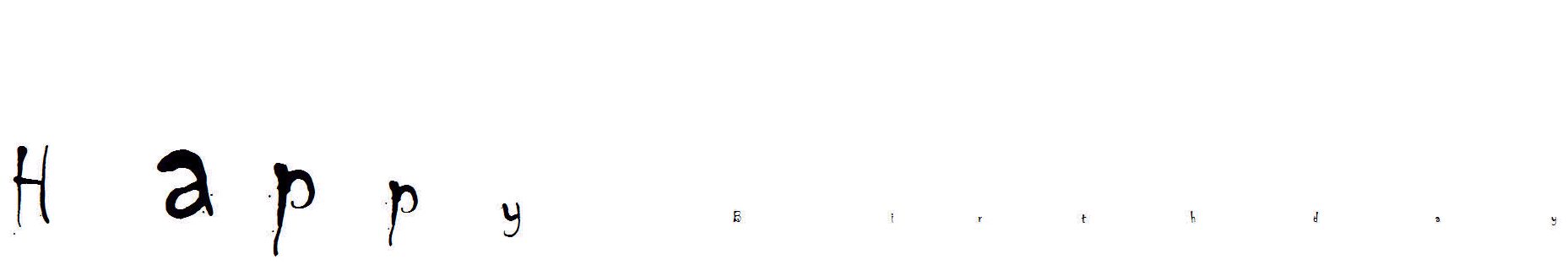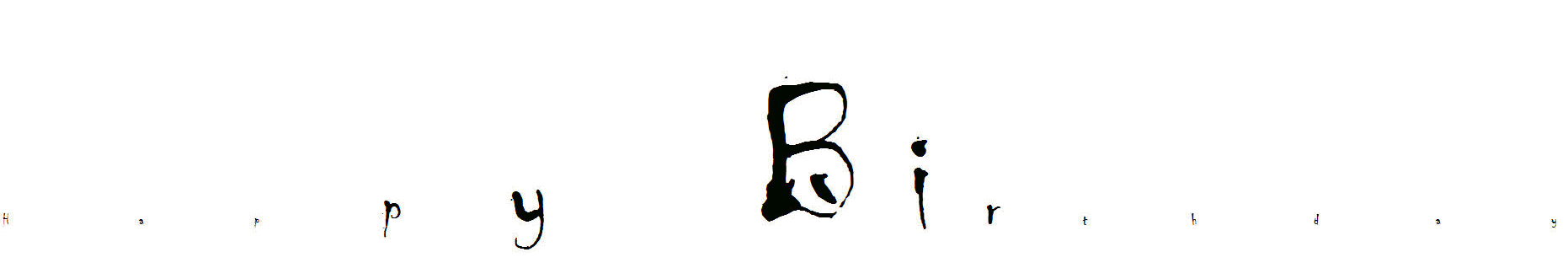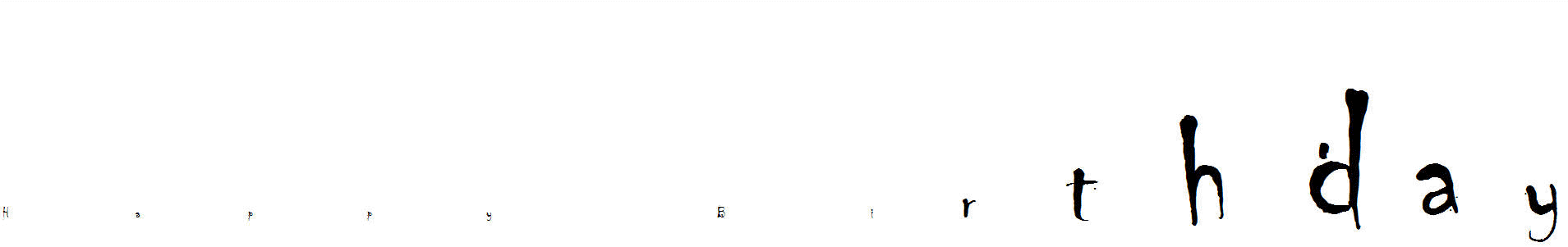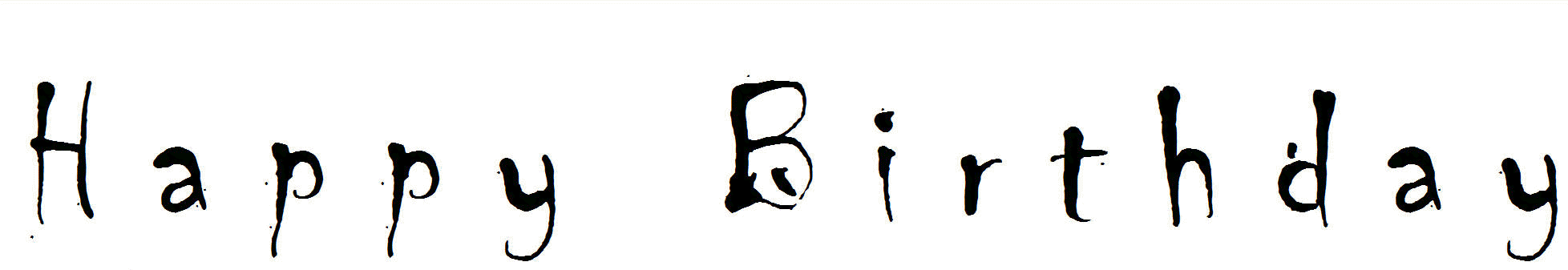﻿ Greeting Wave
You are on the Home/Excel/Templates/Greeting Wave pageShare Your

# Have VB(A) do a greeting in the form of a stadium wave

Not everything in Excel and VBA has to be about work.

Have you seen or participated in a stadium wave (also called an audience wave)?  While not exactly the same, below is VBA code that does a wave using a custom greeting.  The greeting can be just about anything -- a birthday wish, an anniversary greeting, a "get well" message, essentially just about any text message.Put the code below in a standard module and from Excel run the doWave subroutine.  The code opens a new workbook, inserts a new worksheet in it and updates the worksheet.  The workbook is left open when the code finishes.  The only customizable items are in the Const statement at the top of the module.

```Option Explicit
'From Tushar Mehta (www.tushar-mehta.com). This code may be _
used freely provided this comment is left in place.

Const Msg As String = "Happy Birthday", _
MaxSize As Byte = 144, MaxLoops As Byte = 2, _
FontName As String = "Chiller"

Declare Function SetTimer Lib "user32" ( _
ByVal hwnd As Long, ByVal nIDEvent As Long, _
ByVal uElapse As Long, ByVal lpTimerFunc As Long) As Long
Declare Function KillTimer Lib "user32" (ByVal hwnd As Long, _
ByVal nIDEvent As Long) As Long

Dim I As Byte, J As Integer, K As Byte, _
MaxChar As Byte, _
BaseCell As Range, _
TimerID As Long

Private Sub StopTimer()
TimerID = KillTimer(0, TimerID)
BaseCell.Resize(1, MaxChar).Font.Size = MaxSize
Set BaseCell = Nothing
End Sub
Sub TimedProc(ByVal hwnd As Long, _
ByVal uMsg As Long, _
ByVal idEvent As Long, _
ByVal dwTime As Long)
With BaseCell.Offset(0, I - 1)
Dim Mult As Single
Select Case J
Case -7: Mult = 0
Case -6: Mult = 0.25
Case -5: Mult = 0.5
Case -4: Mult = 0.75
Case -3: Mult = 1
Case -2: Mult = 0.75
Case -1: Mult = 0.5
Case -0: Mult = 0.25
End Select
.Offset(0, J).Font.Size = 11 + Mult * (MaxSize - 11)
End With
J = J + 1
If J > 0 Then J = -7: I = I + 1
If I > MaxChar Then I = 1: K = K + 1
If K > MaxLoops Then StopTimer
End Sub
Private Sub Initialize()
Application.ScreenUpdating = False
With BaseCell
.Offset(0, -8).Font.Size = MaxSize
.Offset(0, -8).Resize(1, 8).EntireColumn.Hidden = True
If FontName <> "" Then .EntireRow.Font.Name = FontName
MaxChar = Len(Msg) + 8
For I = 1 To Len(Msg)
BaseCell.Offset(0, I - 1).Value = Mid(Msg, I, 1)
Next I
With .Resize(1, MaxChar - 8)
.Font.Size = MaxSize
.EntireColumn.AutoFit
.Select
With .Parent.Parent.Windows(1)
.Zoom = True
.DisplayGridlines = False
End With
.Font.Size = 11
End With
.Offset(1, 0).Select
End With
Application.ScreenUpdating = True
K = 1
I = 1
J = -7
End Sub
Sub doWave()
Initialize
TimerID = SetTimer(0, 0, 50, AddressOf TimedProc)
End Sub
```